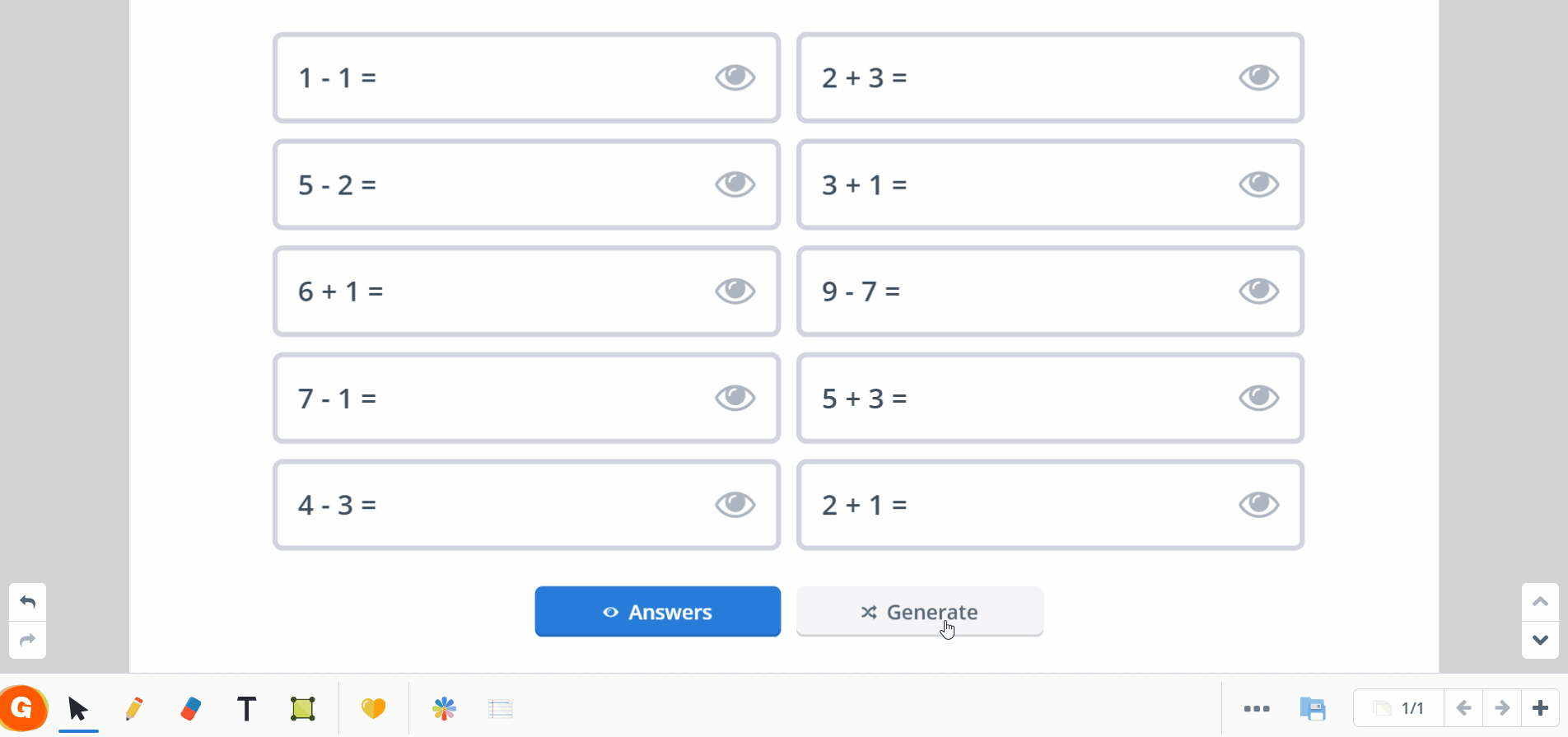# New Tool: The Math Problem Generator

April 15, 2021
0 minutes

With our math problem generator, teachers can quickly create sets of math problems to help students practice addition, subtraction, multiplication, and division. Answers are just a click away, making this a flexible tool that can easily be used throughout the day.## How does it work?

The math problem generator lets educators create custom sets of math problems with answers just a click away. The answers can be revealed one by one (by clicking the eye symbol next to the problem you want to solve) or as a set (by clicking “Answers”). If you want to see a new set of problems, simply click “generate.”Tip: Like with other Gynzy tools, the math problem generator can be easily resized, making it easy to combine with other tools and elements in your lessons.

You determine the types of math problems shown by making a selection in the settings menu. You have multiple options to choose from which allows you to generate math problems for all levels.

On the left side of the settings you find the general options, like the number of problems to show (1, 2, 3, 5, 10, 15 or 20). You also are given extra options that relate to specific math problems, like regrouping within addition and subtraction.

On the right side of the settings you select the type of math problem (addition, subtraction, multiplication, or division). You select the type of problem by setting a checkmark by that type, and select the range in which the math problem may be found (to 10, 100, etc.). You can also choose specific times tables or division tables.

Tip: Instead of a set of math problems, you can also use this tool to show a single math problem. This means you have a quick and easy math problem generator that you can use during a lesson or to focus on a specific skill.

## Math Lessons from Gynzy

The math problem generator is just the latest tool in our Library that helps teachers deliver engaging math lessons to students, no matter where class takes place. While putting together your math lessons, consider trying our fun math games as a way to give students another chance to practice new concepts.

Our math lessons and activities are designed to be used on an in-class interactive whiteboard or on students’ personal devices. Wherever class takes place, Gynzy is the all-in-one educational solution to keep students engaged.